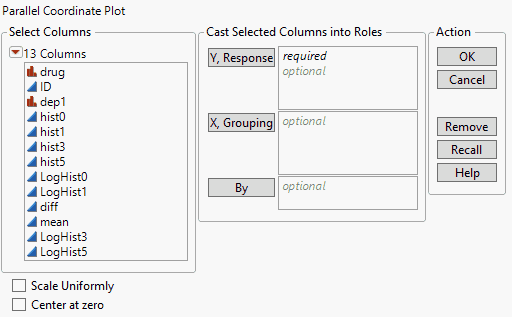Essential Graphing > Parallel Plots > Launch the Parallel Plot Platform
Publication date: 11/10/2021

# Launch the Parallel Plot Platform

Launch the Parallel Plot platform by selecting Graph > Parallel Plot.

Figure 7.4 The Parallel Plot Launch WindowY, Response

Variables appear on the horizontal axis of the parallel plot. These values are plotted and connected in the parallel plot.

X, Grouping

Produces a separate parallel plot for each level of the variable.

By

Identifies a column that creates a report consisting of separate analyses for each level of the specified variable.

Scale Uniformly

Represents all variables on the same scale, adding a y-axis to the plot. Without this option, each variable is on a different scale.

To allow for proper comparisons, select this option if your variables are measured on the same scale.

Center at zero

Centers the parallel plot (not the variables) at zero.

After you click OK, the Parallel plot appears.